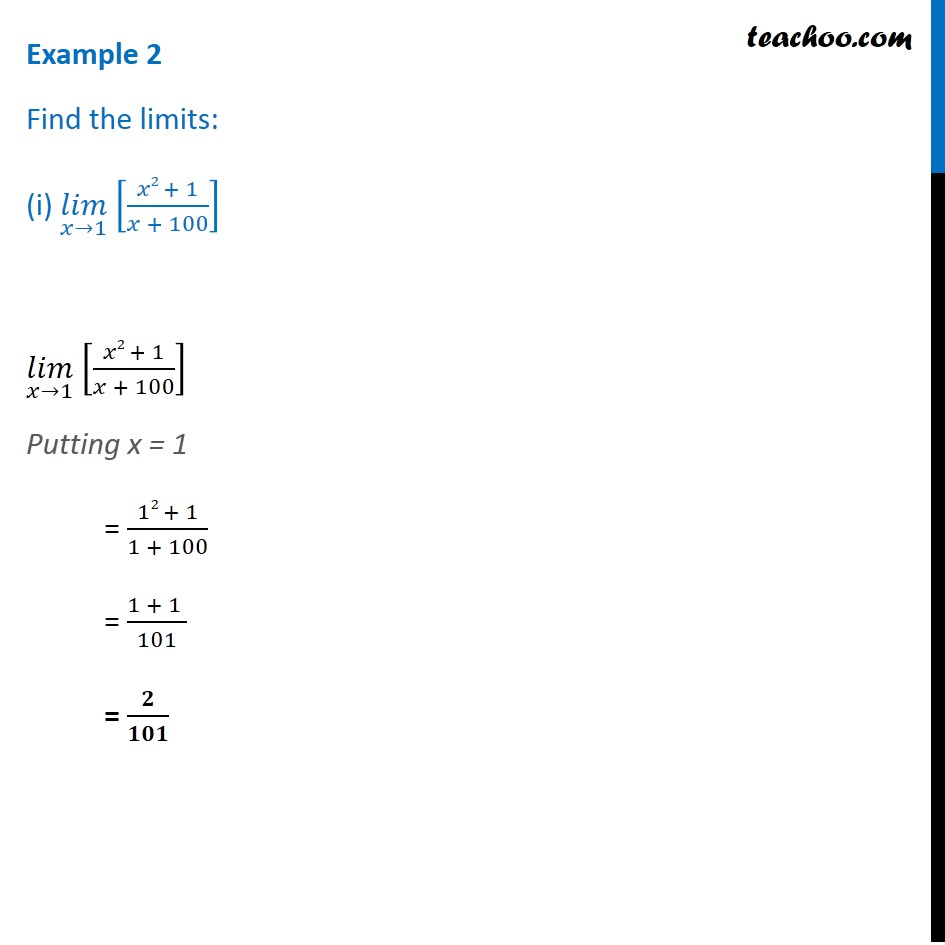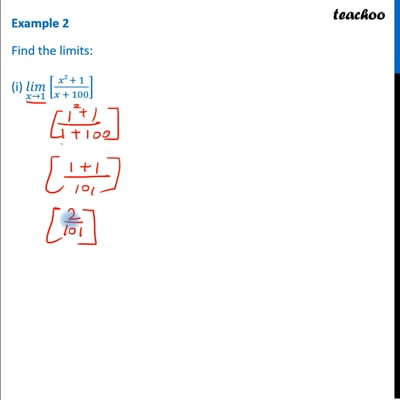Chapter 13 Class 11 Limits and Derivatives

Class 11
Important Questions for exams Class 11This video is only available for Teachoo black users

Get live Maths 1-on-1 Classs - Class 6 to 12

### Transcript

Example 2 Find the limits: (i) (𝑙𝑖𝑚)┬(𝑥→1) [(𝑥2 + 1)/(𝑥 + 100)] (𝑙𝑖𝑚)┬(𝑥→1) [(𝑥2 + 1)/(𝑥 + 100)] Putting x = 1 = (12 + 1)/(1 + 100) = (1 + 1 )/101 = 𝟐/𝟏𝟎𝟏